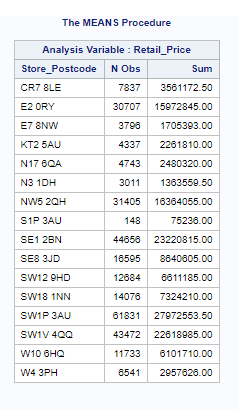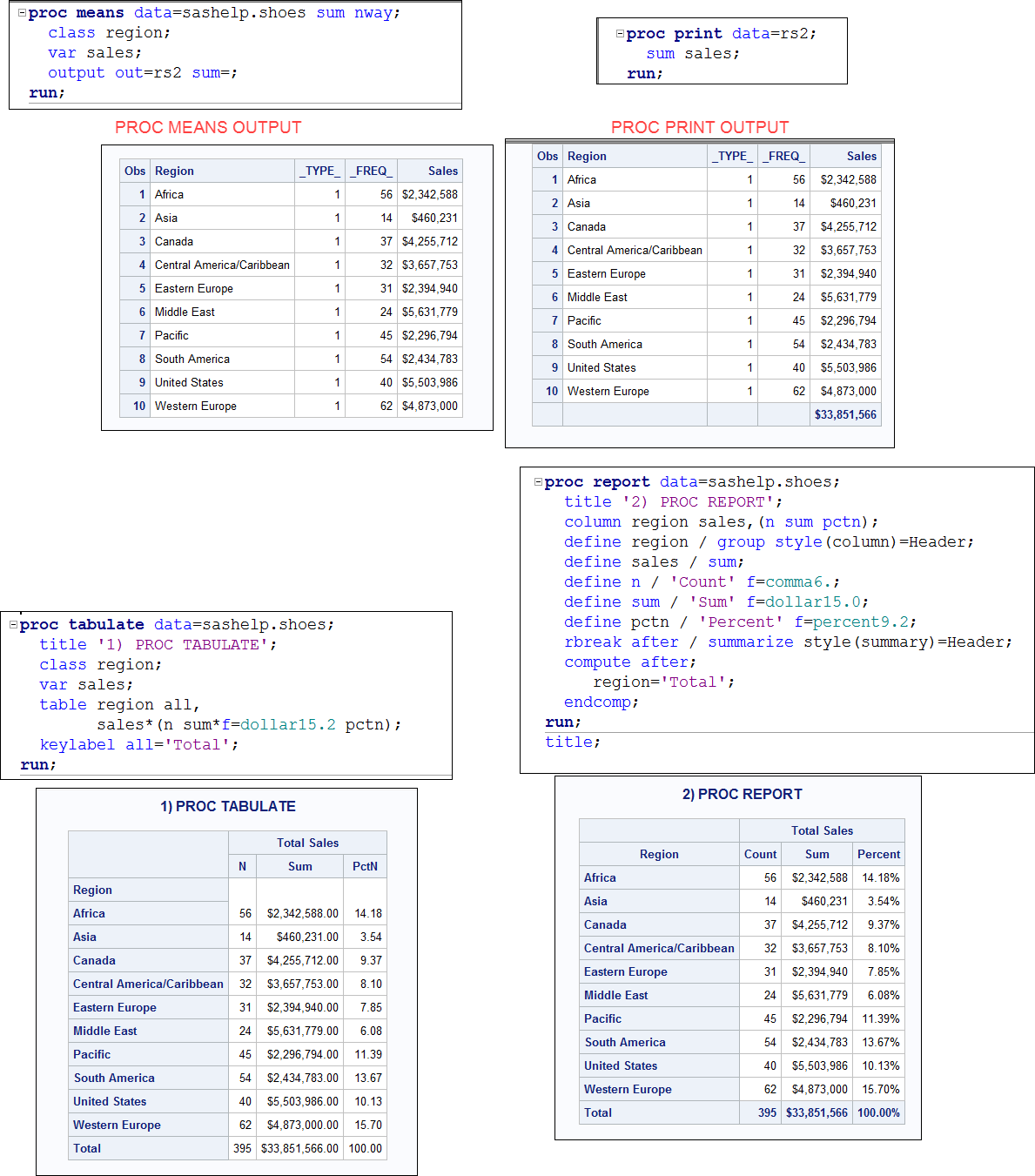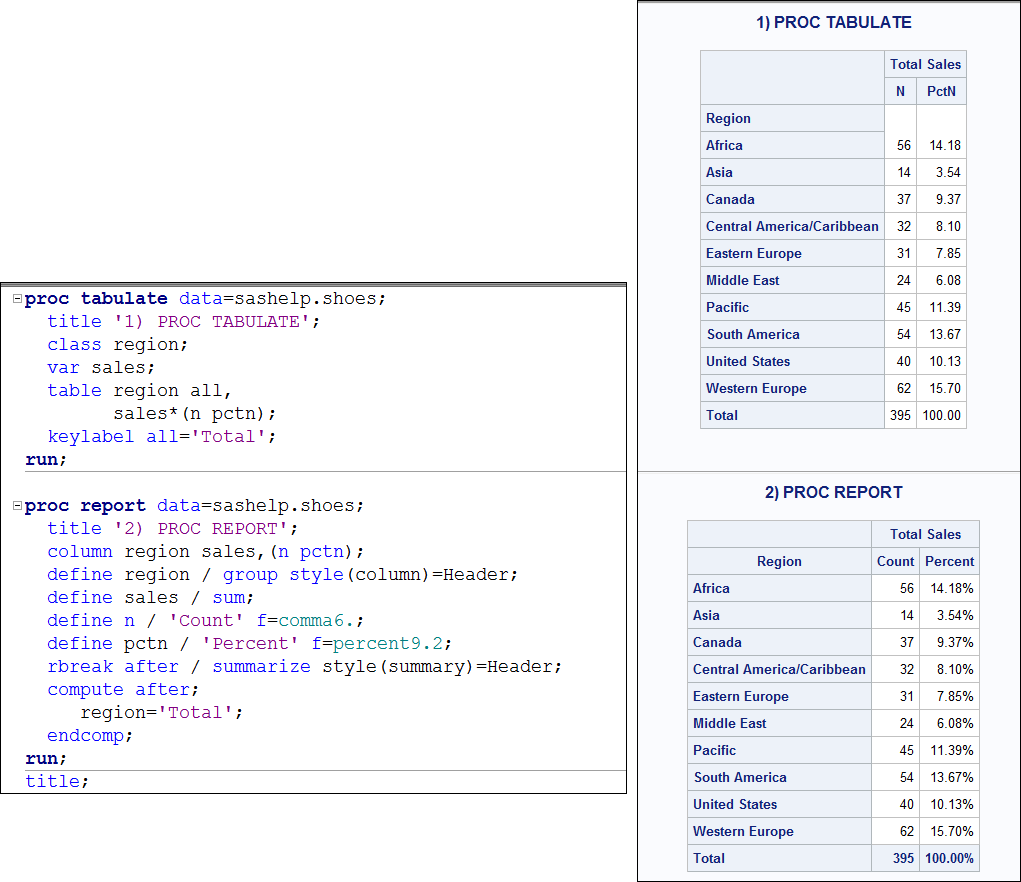## Calculating percentage in proc means or proc report or proc tabulate

Hi All,

I am stucked here:

My code is:

proc means data=final_file_distance sum;
class store_postcode;
var retail_price;
output out=rs2;
run;

Output is attached in attachment.

What /I want is :

(1) to calculate overall sum of " sum" column as total_sale

(2) PErcentage of sale for every StorePostcode   (i.e sum/total_sale)% for individual StorePostcode1 ACCEPTED SOLUTION

Accepted Solutions

## Re: Calculating percentage in proc means or proc report or proc tabulate

Sorry, I don't open Excel files. It is better to post your data in a DATA step program that anyone can read as a text file.

In my example, SASHELP.SHOES has a variable called SALES, in your example, you have a variable called RETAIL_PRICE.

Your PROC MEANS code is giving your results the SUM or RETAIL_PRICE grouped by the class variable STORE_POSTCODE.

In my examples, SALES is the equivalent of your variable RETAIL_PRICE and REGION is the equivalent of STORE_POSTCODE. In SASHELP.SHOES there are multiple sales for every REGION, so both REPORT and TABULATE are summarizing and calculating percent. Notice in the screen shot below (still using SASHELP.SHOES) how the sum of SALES for each region is the same whether you use PROC MEANS, PROC REPORT or PROC TABULATE. Then notice how the grand total of the SALES figure is the same. Notice how the percents calculate by REPORT and TABULATE are the same.

PROC MEANS will provide you with the overall GRAND TOTAL for your RETAIL_PRICE variable, but not in the most usable way possible. READ in the documentation about the use of the _TYPE_ automatic variable. Personally, I find using TABULATE or REPORT much easier than PROC MEANS for sums and percents.

cynthia3 REPLIES 3

## Re: Calculating percentage in proc means or proc report or proc tabulate

Hi:

You do not need to run PROC MEANS first. Either PROC TABULATE or PROC REPORT would give you the percentages you want. You showed the output from PROC MEANS, not your desired output. And, you did not post the input data, but take a look at SASHELP.SHOES -- that has regions and sales. So either of these procedures would work:For what you want to do, either of these procedures can summarize, like PROC MEANS and then calculate the percentage without making an extra pass through the data.

cynthia

## Re: Calculating percentage in proc means or proc report or proc tabulate

Hi,

I am not having anything in my input data which tells me about sum I calculated it by proc measn

The solution you provided contains sales data but in my data I dont have it (rather I have to calculate it)

i am attaching input file in the attachment

## Re: Calculating percentage in proc means or proc report or proc tabulate

Sorry, I don't open Excel files. It is better to post your data in a DATA step program that anyone can read as a text file.

In my example, SASHELP.SHOES has a variable called SALES, in your example, you have a variable called RETAIL_PRICE.

Your PROC MEANS code is giving your results the SUM or RETAIL_PRICE grouped by the class variable STORE_POSTCODE.

In my examples, SALES is the equivalent of your variable RETAIL_PRICE and REGION is the equivalent of STORE_POSTCODE. In SASHELP.SHOES there are multiple sales for every REGION, so both REPORT and TABULATE are summarizing and calculating percent. Notice in the screen shot below (still using SASHELP.SHOES) how the sum of SALES for each region is the same whether you use PROC MEANS, PROC REPORT or PROC TABULATE. Then notice how the grand total of the SALES figure is the same. Notice how the percents calculate by REPORT and TABULATE are the same.

PROC MEANS will provide you with the overall GRAND TOTAL for your RETAIL_PRICE variable, but not in the most usable way possible. READ in the documentation about the use of the _TYPE_ automatic variable. Personally, I find using TABULATE or REPORT much easier than PROC MEANS for sums and percents.

cynthiaDiscussion stats
• 3 replies
• 15336 views
• 0 likes
• 2 in conversation